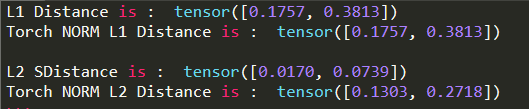# How torch.norm() works? and How it calculates L1 and L2 loss?

I don’t understand how torch.norm() behave and it calculates the L1 loss and L2 loss? When p=1, it calculates the L1 loss, but on p=2 it fails to calculate the L2 loss…

Can somebody explain it?

a, b = torch.rand((2,2)), torch.rand((2,2))

var1 = torch.sum(torch.abs((a * b)), 1)
print("L1 Distance is : ", var1)

var2 = torch.norm(((a * b)), 1, -1)
print("Torch NORM L1 Distance is : ", var2)

var3 = torch.sum(((a * b)) ** 2, 1)
print("L2 SDistance is : ", var3)

var4 = torch.norm(((a * b)), 2, -1)
print("Torch NORM L2 Distance is : ", var4)

And the computed output is as:``````import torch
a, b = torch.rand((2,2)), torch.rand((2,2))

var1 = torch.sum(torch.abs((a * b)), 1)
print("L1 Distance is : ", var1)

var2 = torch.norm(((a * b)), 1, -1)
print("Torch NORM L1 Distance is : ", var2)

var3 = torch.sum(((a * b)) ** 2, 1).sqrt()
print("L2 SDistance is : ", var3)

var4 = torch.norm(((a * b)), 2, -1)
print("Torch NORM L2 Distance is : ", var4)
``````

``````L1 Distance is :  tensor([0.0924, 0.2528])